Question

Part A When titrated with a 0.1038 M solution of sodium hydroxide, a 52.00 mL solution...Part A When titrated with a 0.1038 M solution of sodium hydroxide, a 52.00 mL solution of an unknown polyprotic acid required 22.86 mL to reach the first equivalence point. Calculate the molar concentration of the unknown acid. VALDO ? Submit Request Answer

Balanced chemical equation is:

NaOH + HnA ---> NaHn-1A + H2O

Here:

M(NaOH)=0.1038 M

V(NaOH)=22.86 mL

V(HnA)=52.0 mL

According to balanced reaction:

1*number of mol of NaOH =1*number of mol of HnA

1*M(NaOH)*V(NaOH) =1*M(HnA)*V(HnA)

1*0.1038*22.86 = 1*M(HnA)*52.0

M(HnA) = 0.04563 M

Earn Coins

Coins can be redeemed for fabulous gifts.

Similar Homework Help Questions
• Part A When titrated with a 0.1198 M solution of sodium hydroxide, a 58.00 mL solution...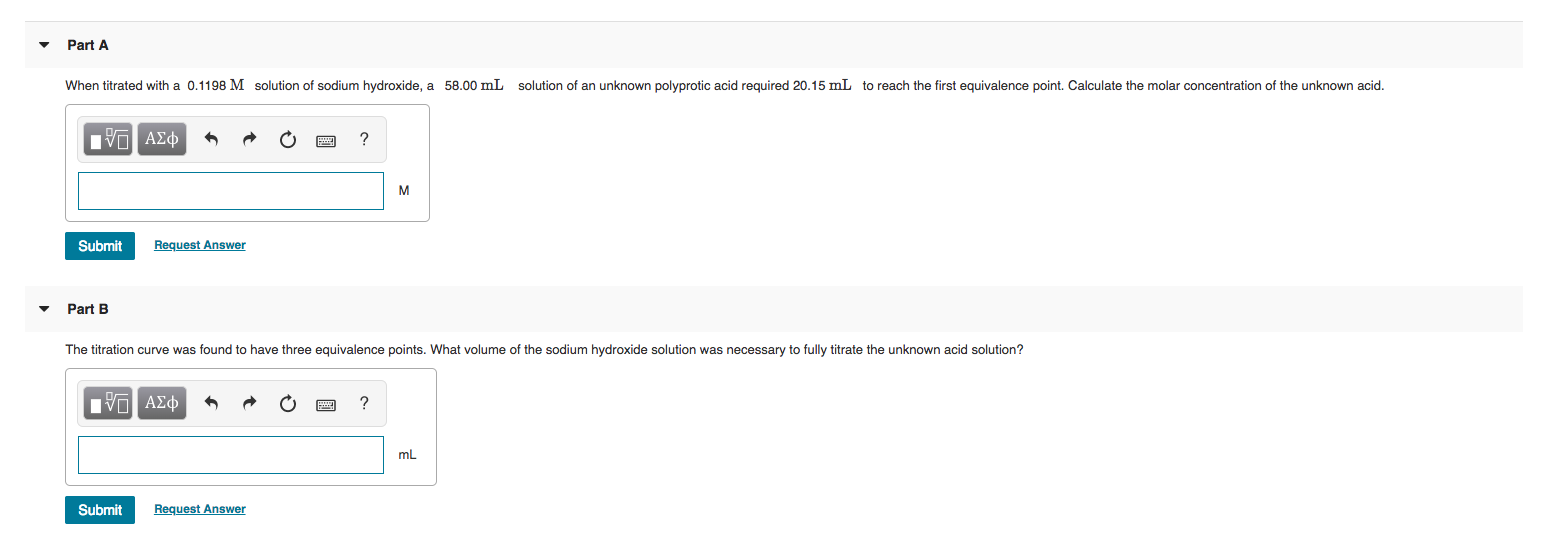Part A When titrated with a 0.1198 M solution of sodium hydroxide, a 58.00 mL solution of an unknown polyprotic acid required 20.15 mL to reach the first equivalence point. Calculate the molar concentration of the unknown acid. O A¢ * R O ? Submit Request Answer Part B The titration curve was found to have three equivalence points. What volume of the sodium hydroxide solution was necessary to fully titrate the unknown acid solution? IVO ADD A O O...

• 2. Calculate the mass of solid sodium hydroxide needed to prepare 300 mL of 0.1 M...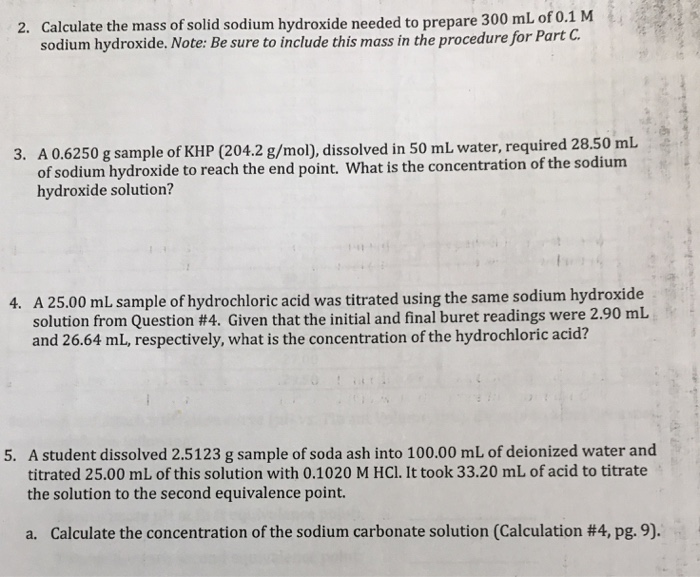2. Calculate the mass of solid sodium hydroxide needed to prepare 300 mL of 0.1 M sodium hydroxide. Note: Be sure to include this mass in the procedure for Part C. 3. A 0.6250 g sample of KHP (204.2 g/mol), dissolved in 50 mL water, required 28.50 mL of sodium hydroxide to reach the end point. What is the concentration of the sodium hydroxide solution? 4. A 25.00 mL sample of hydrochloric acid was titrated using the same sodium hydroxide...

• A 50.0 mL sample of 0.150 M sodium hydroxide is titrated with 0.250 M nitric acid....

A 50.0 mL sample of 0.150 M sodium hydroxide is titrated with 0.250 M nitric acid. Calculate: a. the pH after adding 10.00 mL of HNO3 b. the pH after adding 40.00 mL of HNO3 c. the volume required to reach the equivalence point d. the pH at the equivalence point

• Questions 1. Calculate the molarity of a sodium hydroxide (NaOH) solution that is titrated with 0.6887...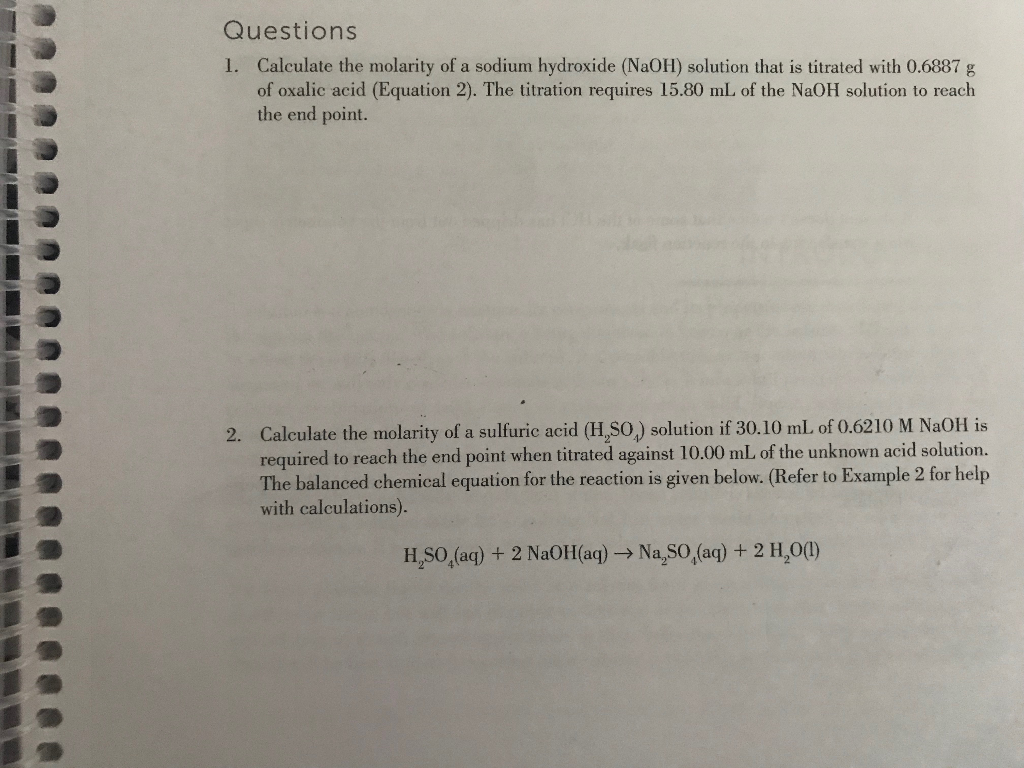Questions 1. Calculate the molarity of a sodium hydroxide (NaOH) solution that is titrated with 0.6887 g of oxalic acid (Equation 2). The titration requires 15.80 mL of the NaOH solution to reach the end point. Calculate the molarity of a sulfuric acid (H,SO) solution if 30.10 mL of 0.62 10 M NaOH is required to reach the end point when titrated against 10.00 mL of the unknown acid solution. The balanced chemical equation for the reaction is given below....

• A sodium hydroxide solution of unknown concentration is titrated against 0.8765 g KHPh (Potassium acid phalate, a monoprotic acid, MW 204.3 g/mol)

1.A sodium hydroxide solution of unknown concentration is titrated against 0.8765 g KHPh (Potassium acid phalate, a monoprotic acid, MW 204.3 g/mol). 48.6 mL of the sodium hydroxide solution is required to reach the endpoint of the titration. What is the concentration of the unknown sodium hydroxide solution. 2.This sodium hydroxide solution is then used to titrate an unknown nitric acid sample. 25.0 mL of the nitric acid solution is titrated. 32.8 mL of the sodium hydroxide solution is required...

• did i do this correctly? a. 15.0 mL of Sulfuric acid wanunknown concentration was titrated! using a 0.450 M Sodium h...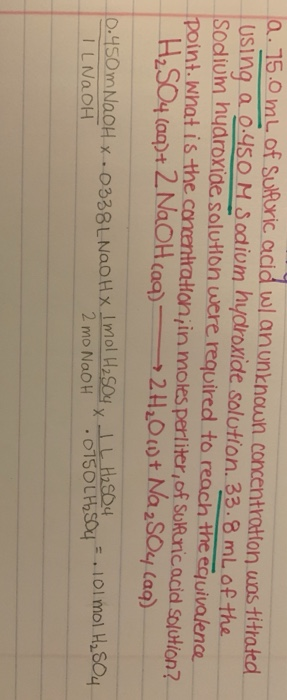did i do this correctly? a. 15.0 mL of Sulfuric acid wanunknown concentration was titrated! using a 0.450 M Sodium hydroxide solution. 33.8 mL of the Sodium hydroxide solution were required to reach the equivalence point. What is the concentration in moles perliter, of sulfuric acid solution? H2SO4 (aq)+ 2NaOH(aq) +2H2011)+ Naz Soy Cag) 10.450m NaOH x .0338LNaOHX M 200 x ILNaOH 2 mo NaOH..0750LH Sou .lol mol H2SO4

• 25.000 mL of sodium hydroxide is titrated with 0.100 M sulfuric acid. It requires 15.71 mL...

25.000 mL of sodium hydroxide is titrated with 0.100 M sulfuric acid. It requires 15.71 mL of acid to reach the end point. Calculate the concentration of the base. A. . 159M B. .0628M c. .126M D. .100M how do you do this problem

• 1) A 25.00 mL sample of HBr is titrated with a 0.150 M standardized sodium hydroxide...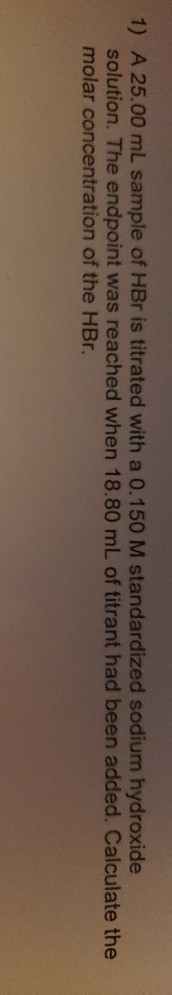1) A 25.00 mL sample of HBr is titrated with a 0.150 M standardized sodium hydroxide solution. The endpoint was reached when 18.80 mL of titrant had been added. Calculate the molar concentration of the HBr.

• When 30.0 mL of an unknown acid was titrated with 0.323 M NaOH

When 30.0 mL of an unknown acid was titrated with 0.323 M NaOH, 33.1 mL of the base was required to reach the equivalence point. What was the concentration of the acid? Please include units in your answer

• When a 23.2 mL sample of a 0.345 M aqueous acetic acid solution is titrated with...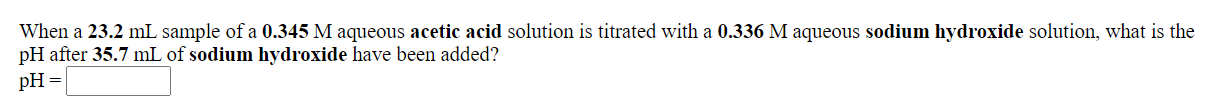When a 23.2 mL sample of a 0.345 M aqueous acetic acid solution is titrated with a 0.336 M aqueous sodium hydroxide solution, what is the pH after 35.7 mL of sodium hydroxide have been added? pH = What is the pH at the equivalence point in the titration of a 27.6 mL sample of a 0.423 M aqueous hydrocyanic acid solution with a 0.431 M aqueous potassium hydroxide solution? pH=